Module 8 - Continuity

Introduction | Lesson 1 | Lesson 2 | Lesson 3 | Self-Test

Lesson 8.3: Piecewise Functions

In this lesson you will modify a piecewise function to make it continuous and then graph the function using Boolean operators.

Consider the function defined by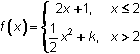8.3.1 By using the definition of continuity, find the value of k that makes the function continuous at

Graphing a Piecewise Function

Display the graph ofusing the value of k that makes the function continuous.

• Set Xres = 1.
• Enter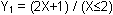You can paste "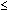" from the Test menu by pressing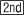[TEST]. [TEST] is above.
• Enter Y2 = ( (1/2)X2+3 ) / (X>2)
The symbol ">" is in the TEST menu.
• Graph the function in a [-3, 7, 1] x [-3, 25, 5] window.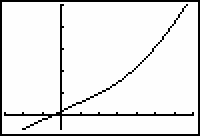The graph appears to be continuous at x = 2.

8.3.2 Describe how the graph would change if you changed k to 7. Click here for the answer.

Illustrate the result of changing the value of k to 7.

• Set Y2=( (1/2)X2+7 ) / (X>2).Editing Existing Expressions Rather than retyping the entire expression in Y2 you can simply replace the "3" with a "7". Move the cursor on to the "3" and press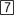. The old value should be replaced since the calculator is in the overwrite mode. The calculator shows it is in the overwrite mode when the cursor is a blinking solid rectangle. It can be changed from overwrite to insert mode and back again by pressing[INS]. ([INS] is abovein the first row of keys.) Each time you press[INS] the mode changes, or "toggles," between overwrite and insert. In insert mode, the cursor is a blinking underline character. Whenever an arrow key or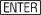is pressed, the calculator returns to overwrite mode.• Display the graph of the function by pressing.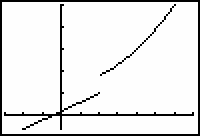The second part of the piecewise function has been shifted upward, and the function is no longer continuous at x = 2. The discontinuity in this modified function (k = 7) is a jump discontinuity.

8.3.3 Evaluate the left- and right-hand limits of the piecewise function with k = 7 at x = 2. Click here for the answer.

< Back | Next >